• Call Now

1800-102-2727•

# Gauss’s Law - Properties of a Gaussian surface, Practice Problems, FAQs

Symmetry is not only beautiful but also very helpful in calculations at times. Sometimes calculating the electric field becomes easy when we know the charge distribution is such that the electric field produced is symmetric about the charge distribution. In such cases the electric field can be calculated by means of Gauss law which requires little calculation! Let’s see how!

Table of content:

• Gauss’s Law
• Properties of a Gaussian surface
• Practice Problems
• FAQs

## Gauss’s Law:

The net flux through a closed surface is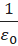times the net charge enclosed by the surface.

From Gauss’s law, the net flux through a surface is given by,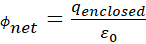Gauss’s law is applicable for any closed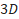surface, independent of its shape and size.

The charge inside is the net charge enclosed by the surface. This is the sum of all charges enclosed.

In case the enclosed surface includes no charge inside, then the net electric flux through the surface is zero.

Gauss’s law is based on the inverse square dependence on the distance as in Coulomb's law.

Any surface has two sides: inwards and outwards. The electric flux going out from the closed surface is taken to be as positive flux and electric flux going inward is taken to be with negative signs.

Gaussian surface:

The Gaussian surface is defined as a closed 3-D surface residing on the periphery of a certain volume where Gauss's law is applied. With the help of the Gaussian surface, we can find the flux of any vector field. The Gaussian surface can be imaginary or real. The shape depends on the type of charge or charge distribution inside the Gaussian surface.here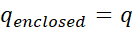Net flux through a surface is given by,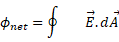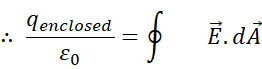## Properties of a Gaussian surface:

Gauss’s law is applicable to any closed 3D surface.

For ease of calculation the electric field must be symmetric and equal in magnitude at all the points on the Gaussian surface. The angle between and dA must be the same at all the points of the Gaussian surface(usually,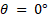or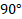).

For a point charge, the Gaussian surface will be a sphere.

For a line charge distribution, the Gaussian surface will be a cylinder.## Practice Problems:

Q1. There are three charges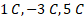inside a sphere. Find the net flux through the sphere.

Ans. The net charge inside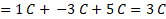So, the flux will be,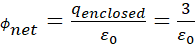Q2. There are four point-charges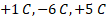and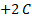. What will be the flux coming out through the surface of an arbitrary geometric shape enclosing the first three point-charges?

Ans. The flux coming out through a surface isSo, the flux coming out through the arbitrary shaped closed surface will be,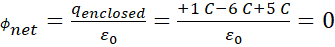Q3. There is a line charge of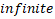length with line charge density of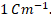What will be the field intensity at a distance of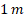from the line?

Ans. Let’s consider a length of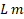. Consider a imaginary cylinder with radiusaround it.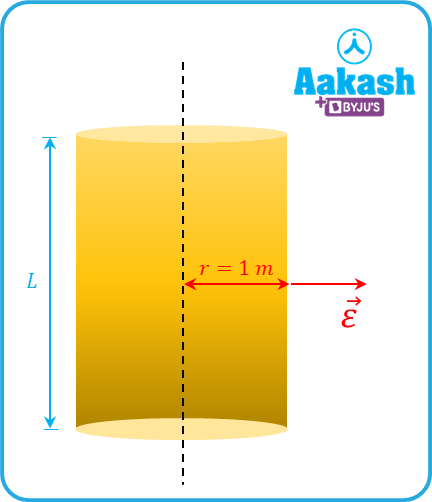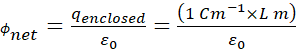Again,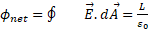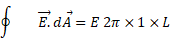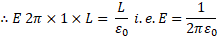Q4. A point charge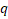is placed at one of the corners of a cube. What is the flux coming out through the faces of the cube?

Ans. Let the point charge is placed at the centre of a cube of side length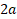.

The cube is actually made of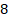small cubes of side length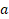.If we just take the small cube into consideration, it will satisfy the problem statement.

The total flux through the big cube is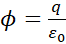The flux through a small cube will be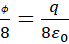## FAQs:

Q1. Does Gauss law work for open surfaces?

Ans. Gauss law is only applicable for closed surfaces. The net charge enclosed by the closed 3D surface is used in calculation of flux through the Gaussian surface.

Q2. How to choose an appropriate Gaussian surface?

Ans. Choosing an appropriate Gaussian surface is important. We should always seek a symmetrical surface with respect to the charge distribution. For example, we should choose a spherical surface when the charge distribution is spherically symmetric. We should choose a cylindrical surface when the charge distribution is cylindrically symmetric.

Q3. Can Gauss law be used to derive Coulomb's law?

Ans. Yes.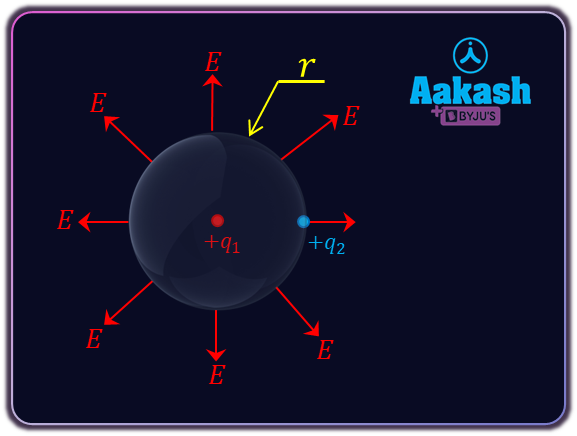Let a point charge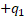is at a distancefrom another point charge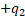.

The electric flux through an imaginary Gaussian surface of spherical shape of radiusis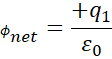The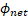can be calculated as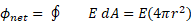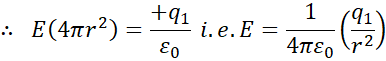The force as experienced by the chargewill be,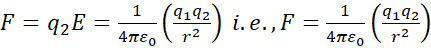which is Coulomb's Law.

Q4. Why we change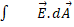to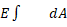in calculation relating to the Gaussian surface?

Ans. Gauss Law is particularly very useful in case the electric field is constant over the Gaussian surface. We are considering it. For example, if the charge distribution is spherically symmetric, we chose a concentric Gaussian surface having a constant electric field in magnitude everywhere on the surface. Thus, we can pull the field,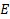out of the integral.Talk to our expert
Resend OTP Timer =
By submitting up, I agree to receive all the Whatsapp communication on my registered number and Aakash terms and conditions and privacy policy